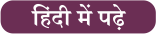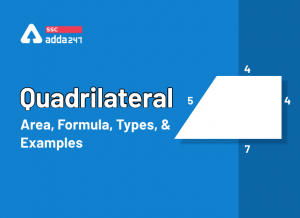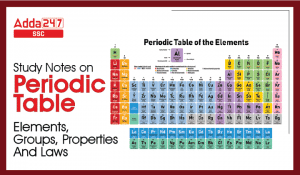Latest SSC jobs   »   Arithmetic Study Notes :   »   Profit and Loss: Notes and Questions

# Profit and Loss Formula, Concept and Study Notes

Profit and Loss: Quantitative Aptitude is one of the most important topics and it is asked in almost every government recruitment exam. Generally, the questions that are questions asked are related to basic concepts and formulas. Generally, the questions are questions asked related to basic concepts and formulas of Profit and Loss. To score full marks on this topic, you must practice enough questions and get acquainted with the concept behind it. We have covered important notes and questions related to Profit and Loss.

## Profit and Loss

Profit(P): The amount gained by selling a product with more than its cost price.

Loss(L): The amount the seller incurs after selling the product less than its cost price is mentioned as a loss.

Cost Price (CP): The price at which an article is purchased is called its cost price (C.P.)

Selling Price (SP): The price at which the article is sold is called its selling price (S.P.)

## Profit and Loss Formula

1. If the cost price (C.P.) of the article is equal to the selling price (S.P.), then there is no loss or gain.
2. If the selling price (S.P.) > cost price (C.P.), then the seller is said to have a profit or gain. Gain/Profit = S.P. – C.P.
3. If the cost price (C.P.) > selling price (S.P.), then the seller is said to have a loss. Loss = C.P. – S.P.
4. Gain % = (Gain × 100)/(C.P.)
5. Loss% = (Loss × 100)/(C.P.)
6. When the selling price and gain percent are given: C.P.= (100/(100+Gain%))×S.P.
7. When the cost and gain percent are given: S.P=((100+Gain%)/100)×C.P.
8. When the cost and loss percent are given: S.P.=((100-Loss%)/100)×C.P
9. When the selling price and loss percent are given: C.P=(100/(100-Loss%))×S.P
10. If a man buys x items for Rs. y and sells z items for Rs. w, then the gain or loss percent made by him is given by: (xw/zy-1)×100%
11. If the cost price of m articles is equal to the selling price of n articles, then % gain or loss = ((m – n)/n) × 100 (If m > n, it is % gain, and if m < n, it is % loss)
12. If an article is sold at a price S.P.₁, then % gain or % loss is x and if it is sold at a price S.P.₂, then % gain or % loss is y. If the cost price of the article is C.P., then
(S.P₁)/(100+x)=(S.P₂)/(100+y)=(C.P.)/100=(S.P_1-S.P_2)/(x-y); Where x or y is –ve, if it indicates a loss, otherwise it is +ve.
13. If ‘A’ sells an article to ‘B’ at a gain/loss of m% and ‘B’ sells it to ‘C’ at a gain/loss of n%. If ‘C’ pays Rs. z for it to ‘B’ then the cost price for ‘A’ is

#### Click Here to Attempt More Questions From Quantitative Aptitude For SSC CGL

14. If ‘A’ sells an article to ‘B’ at a gain/loss of m% and ‘B’ sells it to ‘C’ at a gain/loss of n%, then the resultant profit/loss percent is given by (m+n+mn/100), where m or n is –ve, if it indicates a loss, otherwise it is +ve.
15. When two different articles are sold at the same selling price, getting a gain/loss of x% on the first and a gain/loss of y% on the second, then the overall% gain or % loss in the transaction is given by
The above expression represents overall gain or loss accordingly as its sign is +ve or –ve.

16. When two different articles are sold at the same selling price getting a gain of x% on the first and loss of x% on the second, then the overall% loss in the transaction is given by (x/10)² %. (Note: In such questions, there is always a loss.)

17. A merchant uses faulty measures and sells his goods at a gain/loss of x%. The overall % gain/loss(g) is given by (100+g)/(100+x)=(True measure)/(Faulty measure). (Note: If the merchant sells his goods at cost price, then x = 0.)

18. A merchant uses y% less weight/length and sells his goods at a gain/loss of x%. The overall % gain/loss is given by [((y+x)/(100-y))×100]%

19. A person buys two items for Rs. A and sells one at a loss of l% and the other at a gain of g%. If each item was sold at the same price, then

(a) The cost price of the item sold at a loss

(b) The cost price of the item sold at a gain

20. If two successive discounts on an article are m% and n%, respectively, then a single discount equivalent to the two successive discounts will be (m+n-mn/100)%

21. If three successive discounts on an article are l%, m%, and n%, respectively, then a single discount equivalent to the three successive discounts will be

18. A shopkeeper sells an item at Rs. z after giving a discount of d% on the labeled price. Had he not given the discount, he would have earned a profit of p% on the cost price. The cost price of each item is given by

## Profit and Loss Questions

Here are some profit and loss examples which will help candidates for understanding the profit and loss properly and according to the exam point of view. Candidates must try to solve thes profit and loss examples given below and verify your answers.

Q1. How much percent more than the cost price should a shopkeeper mark his goods so that after allowing a discount of 25% on the marked price, he gains 20%?
A.  60%
B.  55%
C.  70%
D.  50%
Ans(A)
Solution: Let the cost price of goods be Rs 100.
Gain = 20%
Therefore, Selling price = Rs 120
Discount = 25%
Marked Price = (100/100-25)x120 = Rs. 160 = 60% more
Q2. A dishonest dealer professes to sell his goods at the cost price but uses a false weight of 850 g instead of 1 kg. His gain percent is?
A.  71 11/17%
B.  11 11/17%
C.  17 12/17%
D.  17 11/17%
Ans. (D)
Solution: If a trader professes to sell his goods at cost price, but uses false weights, then
Gain% = {Error/(True value – Error) x 100}%
In the given question, Error = 1000 – 850 = 150
Thus, Gain% = {150/(1000 – 150) x 100}% = 17 11/17%
Q3. An article is sold at a 10% loss. If the selling price is Rs. 40 more, there will be a gain of 15%. The cost price of the article is:
A.  Rs. 140
B.  Rs. 120
C.  Rs. 175
D.  Rs. 160
Ans (D)
Solution: Let the cost price be Rs. x.

Selling Price at 10% loss = 90x/100
Selling Price at 15% gain = 115x/100
Thus, according to the problem,
115x/100 – 90x/100 = 40
x = Rs.160

Q4. The cost price of 20 articles is the same as the selling price of x articles. If the profit is 25%, find out the value of x
A. 15
B. 25
C. 18
D. 16
Ans (D)
Solution: Let the Cost Price (CP) of one article = 1
=> CP of x articles = x (Equation 1)
CP of 20 articles = 20
Given that the cost price of 20 articles is the same as the selling price of x articles
=> Selling price (SP) of x articles = 20 (Equation 2)
Given that Profit = 25%
(SP-CP/CP)=25/100=1/4 ( Equation 3)
Substituting equations 1 and 2 in equation 3,
(20-x)/x=1/4
80-4x=x
5x=80
x=80/5=16
Q5. In a certain store, the profit is 320% of the cost. If the cost increases by 25% but the selling price remains constant, approximately what percentage of the selling price is the profit?
A. 30%
B. 70%
C. 100%
D. 250%

Ans (B)

Solution: Let C.P.= Rs. 100. Then, Profit = Rs. 320, S.P. = Rs. 420.
New C.P. = 125% of Rs. 100 = Rs. 125
New S.P. = Rs. 420.
Profit = Rs. (420 – 125) = Rs. 295.
Required percentage = (295/420 x 100)% = 1475/21 % = 70% (approximately).Compound Interest Formula

SSC CGL Syllabus 2023

SSC CGL Salary 2023

SSC CGL Previous Year Question Paper

## FAQs

### Q. What is profit and loss?

Ans: The amount gained by selling a product with more than the cost price is profit and less than the cost price is loss.

### Q. What is the formula for profit and loss?

Ans: The profit or gain is equal to the selling price minus the cost price. Loss is equal to cost price minus selling price.

### Q. What is the formula of CP and SP?

Ans: CP = ( SP * 100 ) / ( 100 + percentage profit)

#### Congratulations!General Awareness & Science Capsule PDFIncorrect details? Fill the form again here

General Awareness & Science Capsule PDF

Thank You, Your details have been submitted we will get back to you.

### TOPICS:

•Pronouns: Types, Rules And Examples
•Quadrilateral Area, Formula, Types, Prop...
•Periodic Table: Elements, Groups, Proper...
•Mixture and Alligation, Notes, Methods a...
•Speed, Time And Distance Tricks and Ques...
•Important lakes of India | List of Larg...